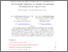Increase the visibility of your scientific production by authorizing the export of your publications to HAL!

# Self-similar solutions with compactly supported profile of some nonlinear Schrödinger equations

Bégout, Pascal and Diaz, Jesus Ildefonso (2014) Self-similar solutions with compactly supported profile of some nonlinear Schrödinger equations. Electronic Journal of Differential Equations, 2014 (90). pp. 1-15.Preview
Text

## Abstract

\textit{Sharp localized}'' solutions (\textit{i.e.} with compact support for each given time $t)$ of a singular nonlinear type Schrödinger equation in the whole space $\R^N$ are constructed here under the assumption that they have a self-similar structure. It requires the assumption that the external forcing term satisfies that $\f(t,x)=t^{-(\vp-2)/2}\F(t^{-1/2}x)$ for some complex exponent $\vp$ and for some profile function $\F$ which is assumed to be with compact support in $\R^N.$ We show the existence of solutions of the form $\vu(t,x)=t^{\vp/2}\U(t^{-1/2}x),$ with a profile $\U,$ which also has compact support in $\R^N.$ The proof of the localization of the support of the profile $\U$ uses some suitable energy method applied to the stationary problem satisfied by $\U$ after some unknown transformation.

Item Type: Article English 2 April 2014 Yes nonlinear self-similar Schrödinger equation, compact support, energy method G- MATHEMATIQUES Institut de mathématiques de Toulouse UT1 19 May 2020 12:11 19 May 2020 12:15 http://publications.ut-capitole.fr/id/eprint/34895

### Available Versions of this ItemView Item